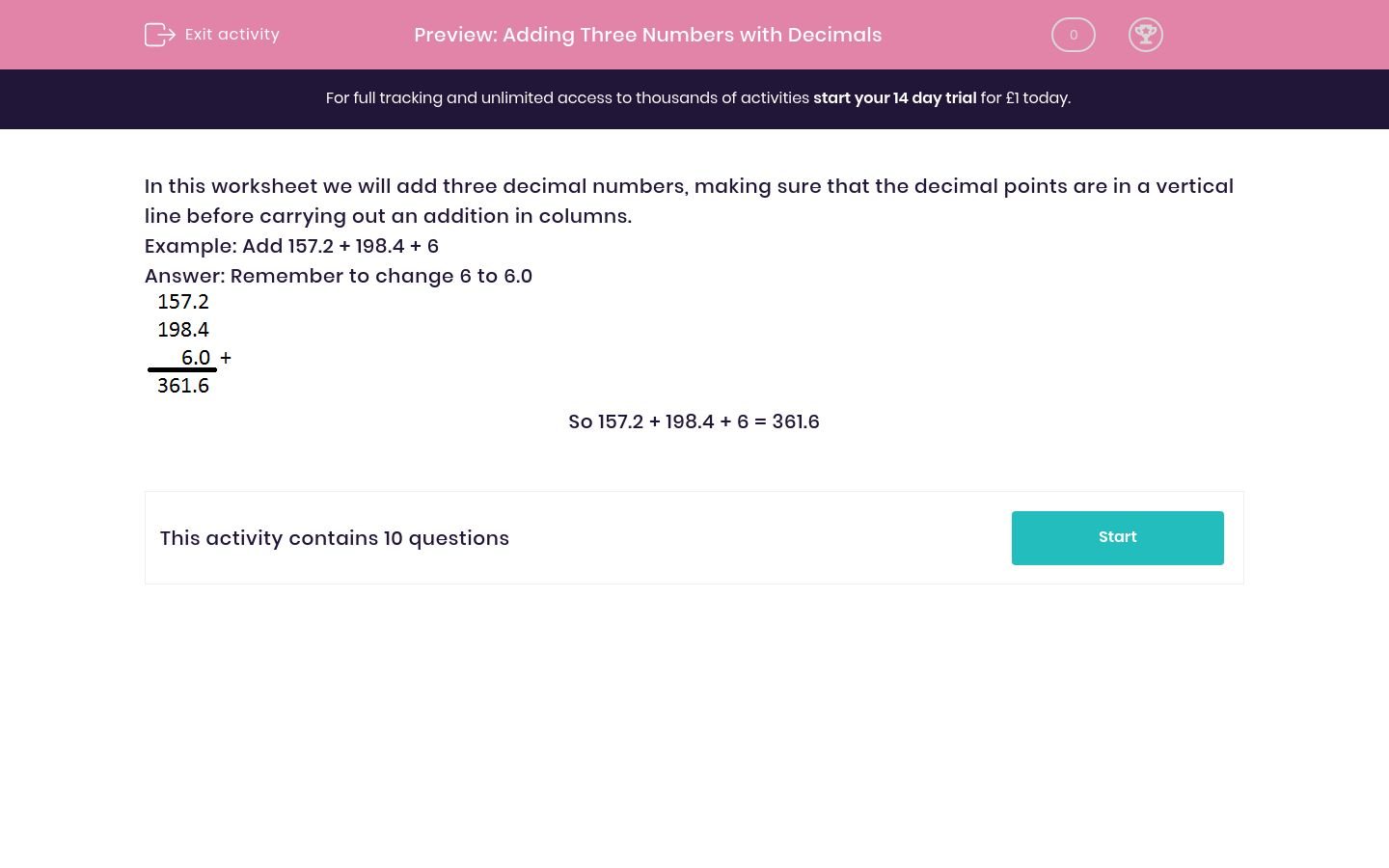# Adding Three Numbers with Decimals

In this worksheet, students add together three numbers, some of which have decimals, using columns and lining up decimals points.Key stage:  KS 2

Curriculum topic:   Maths and Numerical Reasoning

Curriculum subtopic:   Decimals

Difficulty level:### QUESTION 1 of 10

In this worksheet we will add three decimal numbers, making sure that the decimal points are in a vertical line before carrying out an addition in columns.

Example: Add 157.2 + 198.4 + 6

Answer: Remember to change 6 to 6.0So 157.2 + 198.4 + 6 = 361.6

Find the total of

157.2 + 198.4 + 24.9 = _______

Find the total of

157.2 + 6 + 24.9 = _______

Find the total of

198.4 + 6 + 24.9 = _______

Find the total of

157.2 + 198.4 + 99.3 =________

Find the total of

157.2 + 6 + 99.3 = ________

Find the total of

198.4 + 6 + 99.3 = _________

Find the total of

157.2 + 24.9 + 99.3 = ________

Find the total of

198.4 + 24.9 + 99.3 = _______

Find the total of

6 + 24.9 + 99.3 = _______

Find the total of

33.1 + 159.3 + 52 = ________

• Question 1

Find the total of

157.2 + 198.4 + 24.9 = _______

380.5
• Question 2

Find the total of

157.2 + 6 + 24.9 = _______

188.1
• Question 3

Find the total of

198.4 + 6 + 24.9 = _______

229.3
• Question 4

Find the total of

157.2 + 198.4 + 99.3 =________

454.9
• Question 5

Find the total of

157.2 + 6 + 99.3 = ________

262.5
• Question 6

Find the total of

198.4 + 6 + 99.3 = _________

303.7
• Question 7

Find the total of

157.2 + 24.9 + 99.3 = ________

281.4
• Question 8

Find the total of

198.4 + 24.9 + 99.3 = _______

322.6
• Question 9

Find the total of

6 + 24.9 + 99.3 = _______

130.2
• Question 10

Find the total of

33.1 + 159.3 + 52 = ________

244.4
---- OR ----

Sign up for a £1 trial so you can track and measure your child's progress on this activity.

### What is EdPlace?

We're your National Curriculum aligned online education content provider helping each child succeed in English, maths and science from year 1 to GCSE. With an EdPlace account you’ll be able to track and measure progress, helping each child achieve their best. We build confidence and attainment by personalising each child’s learning at a level that suits them.

Get started